Search by Topic

Resources tagged with Working systematically similar to Six Times Five:

Filter by: Content type:
Age range:
Challenge level:

There are 127 results

Broad Topics > Using, Applying and Reasoning about Mathematics > Working systematicallyAge 7 to 14 Challenge Level:

I added together some of my neighbours' house numbers. Can you explain the patterns I noticed?Football Sum

Age 11 to 14 Challenge Level:

Find the values of the nine letters in the sum: FOOT + BALL = GAMEAge 11 to 14 Challenge Level:

Replace the letters with numbers to make the addition work out correctly. R E A D + T H I S = P A G ECinema Problem

Age 11 to 14 Challenge Level:

A cinema has 100 seats. Show how it is possible to sell exactly 100 tickets and take exactly £100 if the prices are £10 for adults, 50p for pensioners and 10p for children.Two and Two

Age 11 to 14 Challenge Level:

How many solutions can you find to this sum? Each of the different letters stands for a different number.How Old Are the Children?

Age 11 to 14 Challenge Level:

A student in a maths class was trying to get some information from her teacher. She was given some clues and then the teacher ended by saying, "Well, how old are they?"Cayley

Age 11 to 14 Challenge Level:

The letters in the following addition sum represent the digits 1 ... 9. If A=3 and D=2, what number is represented by "CAYLEY"?LCM Sudoku

Age 14 to 16 Challenge Level:

Here is a Sudoku with a difference! Use information about lowest common multiples to help you solve it.A First Product Sudoku

Age 11 to 14 Challenge Level:

Given the products of adjacent cells, can you complete this Sudoku?Multiply the Addition Square

Age 11 to 14 Challenge Level:

If you take a three by three square on a 1-10 addition square and multiply the diagonally opposite numbers together, what is the difference between these products. Why?Diagonal Product Sudoku

Age 11 to 16 Challenge Level:

Given the products of diagonally opposite cells - can you complete this Sudoku?Product Sudoku

Age 11 to 14 Challenge Level:

The clues for this Sudoku are the product of the numbers in adjacent squares.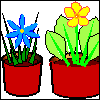More Plant Spaces

Age 7 to 14 Challenge Level:

This challenging activity involves finding different ways to distribute fifteen items among four sets, when the sets must include three, four, five and six items.Cuboids

Age 11 to 14 Challenge Level:

Find a cuboid (with edges of integer values) that has a surface area of exactly 100 square units. Is there more than one? Can you find them all?Reach 100

Age 7 to 14 Challenge Level:

Choose four different digits from 1-9 and put one in each box so that the resulting four two-digit numbers add to a total of 100.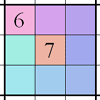Integrated Product Sudoku

Age 11 to 16 Challenge Level:

This Sudoku puzzle can be solved with the help of small clue-numbers on the border lines between pairs of neighbouring squares of the grid.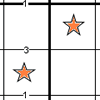Constellation Sudoku

Age 14 to 18 Challenge Level:

Special clue numbers related to the difference between numbers in two adjacent cells and values of the stars in the "constellation" make this a doubly interesting problem.Summing Consecutive Numbers

Age 11 to 14 Challenge Level:

15 = 7 + 8 and 10 = 1 + 2 + 3 + 4. Can you say which numbers can be expressed as the sum of two or more consecutive integers?Factor Lines

Age 7 to 14 Challenge Level:

Arrange the four number cards on the grid, according to the rules, to make a diagonal, vertical or horizontal line.Special Numbers

Age 11 to 14 Challenge Level:

My two digit number is special because adding the sum of its digits to the product of its digits gives me my original number. What could my number be?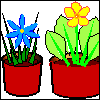More Children and Plants

Age 7 to 14 Challenge Level:

This challenge extends the Plants investigation so now four or more children are involved.A Long Time at the Till

Age 14 to 18 Challenge Level:

Try to solve this very difficult problem and then study our two suggested solutions. How would you use your knowledge to try to solve variants on the original problem?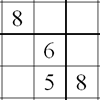Twin Line-swapping Sudoku

Age 14 to 16 Challenge Level:

A pair of Sudoku puzzles that together lead to a complete solution.More Magic Potting Sheds

Age 11 to 14 Challenge Level:

The number of plants in Mr McGregor's magic potting shed increases overnight. He'd like to put the same number of plants in each of his gardens, planting one garden each day. How can he do it?Peaches Today, Peaches Tomorrow...

Age 11 to 14 Challenge Level:

Whenever a monkey has peaches, he always keeps a fraction of them each day, gives the rest away, and then eats one. How long could he make his peaches last for?Ben's Game

Age 11 to 14 Challenge Level:

Ben passed a third of his counters to Jack, Jack passed a quarter of his counters to Emma and Emma passed a fifth of her counters to Ben. After this they all had the same number of counters.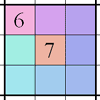Integrated Sums Sudoku

Age 11 to 16 Challenge Level:

The puzzle can be solved with the help of small clue-numbers which are either placed on the border lines between selected pairs of neighbouring squares of the grid or placed after slash marks on. . . .Star Product Sudoku

Age 11 to 16 Challenge Level:

The puzzle can be solved by finding the values of the unknown digits (all indicated by asterisks) in the squares of the $9\times9$ grid.LCM Sudoku II

Age 11 to 18 Challenge Level:

You are given the Lowest Common Multiples of sets of digits. Find the digits and then solve the Sudoku.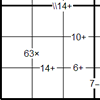Bochap Sudoku

Age 11 to 16 Challenge Level:

This Sudoku combines all four arithmetic operations.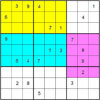Colour Islands Sudoku 2

Age 11 to 18 Challenge Level:

In this Sudoku, there are three coloured "islands" in the 9x9 grid. Within each "island" EVERY group of nine cells that form a 3x3 square must contain the numbers 1 through 9.Multiples Sudoku

Age 11 to 14 Challenge Level:

Each clue in this Sudoku is the product of the two numbers in adjacent cells.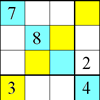Rectangle Outline Sudoku

Age 11 to 16 Challenge Level:

Each of the main diagonals of this sudoku must contain the numbers 1 to 9 and each rectangle width the numbers 1 to 4.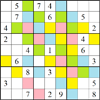Rainstorm Sudoku

Age 14 to 16 Challenge Level:

Use the clues about the shaded areas to help solve this sudokuSticky Numbers

Age 11 to 14 Challenge Level:

Can you arrange the numbers 1 to 17 in a row so that each adjacent pair adds up to a square number?Difference Dynamics

Age 14 to 18 Challenge Level:

Take three whole numbers. The differences between them give you three new numbers. Find the differences between the new numbers and keep repeating this. What happens?American Billions

Age 11 to 14 Challenge Level:

Play the divisibility game to create numbers in which the first two digits make a number divisible by 2, the first three digits make a number divisible by 3...Tea Cups

Age 7 to 14 Challenge Level:

Place the 16 different combinations of cup/saucer in this 4 by 4 arrangement so that no row or column contains more than one cup or saucer of the same colour.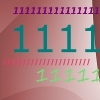Ones Only

Age 11 to 14 Challenge Level:

Find the smallest whole number which, when mutiplied by 7, gives a product consisting entirely of ones.Coins

Age 11 to 14 Challenge Level:

A man has 5 coins in his pocket. Given the clues, can you work out what the coins are?Window Frames

Age 5 to 14 Challenge Level:

This task encourages you to investigate the number of edging pieces and panes in different sized windows.9 Weights

Age 11 to 14 Challenge Level:

You have been given nine weights, one of which is slightly heavier than the rest. Can you work out which weight is heavier in just two weighings of the balance?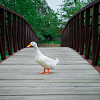Crossing the Bridge

Age 11 to 14 Challenge Level:

Four friends must cross a bridge. How can they all cross it in just 17 minutes?Number Daisy

Age 11 to 14 Challenge Level:

Can you find six numbers to go in the Daisy from which you can make all the numbers from 1 to a number bigger than 25?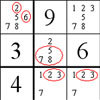The Naked Pair in Sudoku

Age 7 to 16

A particular technique for solving Sudoku puzzles, known as "naked pair", is explained in this easy-to-read article.Age 11 to 16 Challenge Level:

Four numbers on an intersection that need to be placed in the surrounding cells. That is all you need to know to solve this sudoku.Pole Star Sudoku 2

Age 11 to 16 Challenge Level:

This Sudoku, based on differences. Using the one clue number can you find the solution?Consecutive Negative Numbers

Age 11 to 14 Challenge Level:

Do you notice anything about the solutions when you add and/or subtract consecutive negative numbers?More on Mazes

Age 7 to 14

There is a long tradition of creating mazes throughout history and across the world. This article gives details of mazes you can visit and those that you can tackle on paper.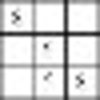Twin Corresponding Sudokus II

Age 11 to 16 Challenge Level:

Two sudokus in one. Challenge yourself to make the necessary connections.